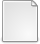# A Schur--Pad\'e Algorithm for Fractional Powers of a Matrix

Higham, Nicholas J. and Lin, Lijing (2011) A Schur--Pad\'e Algorithm for Fractional Powers of a Matrix. SIAM J. on Matrix Analysis and Applications, 32 (3). pp. 1056-1078. ISSN 0895-4798PDF hili11a.pdf Download (1MB)Other powerm_pade.zip Download (3kB)

## Abstract

A new algorithm is developed for computing arbitrary real powers $A^p$ of a matrix $A\in\mathbb{C}^{n\times n}$. The algorithm starts with a Schur decomposition, takes $k$ square roots of the triangular factor $T$, evaluates an $[m/m]$ Pad\'e approximant of $(1-x)^p$ at $I - T^{1/2^k}$, and squares the result $k$ times. The parameters $k$ and $m$ are chosen to minimize the cost subject to achieving double precision accuracy in the evaluation of the Pad\'e approximant, making use of a result that bounds the error in the matrix Pad\'e approximant by the error in the scalar Pad\'e approximant with argument the norm of the matrix. The Pad\'e approximant is evaluated from the continued fraction representation in bottom-up fashion, which is shown to be numerically stable. In the squaring phase the diagonal and first superdiagonal are computed from explicit formulae for $T^{p/2^j}$, yielding increased accuracy. Since the basic algorithm is designed for $p\in(-1,1)$, a criterion for reducing an arbitrary real $p$ to this range is developed, making use of bounds for the condition number of the $A^p$ problem. How best to compute $A^k$ for a negative integer $k$ is also investigated. In numerical experiments the new algorithm is found to be superior in accuracy and stability to several alternatives, including the use of an eigendecomposition and approaches based on the formula $A^p = \exp(p\log(A))$.

Item Type: Article matrix power, matrix root, fractional power, primary matrix function, Schur decomposition, Pad\'e approximation, Pad\'e approximant, matrix logarithm, matrix exponential, MATLAB, CICADA MSC 2010, the AMS's Mathematics Subject Classification > 15 Linear and multilinear algebra; matrix theoryMSC 2010, the AMS's Mathematics Subject Classification > 65 Numerical analysis Nick Higham 05 Oct 2011 20 Oct 2017 14:12 http://eprints.maths.manchester.ac.uk/id/eprint/1677

### Available Versions of this ItemView Item